# Samacheer Kalvi 10th Maths Solutions Chapter 4 Geometry Ex 4.1

## Tamilnadu Samacheer Kalvi 10th Maths Solutions Chapter 4 Geometry Ex 4.1

10th Maths Geometry Exercise 4.1 Solutions Question 1.
Check whether the which triangles are similar and find the value of x.Solution:x = $$\frac{15}{6}$$ = 2.5

10th Maths Exercise 4.1 Question 2.
A girl looks the reflection of the top of the lamp post on the mirror which is 6.6 m away from the foot of the lamppost. The girl whose height is 1.25 m is standing 2.5 m away from the mirror. Assuming the mirror is placed on the ground facing the sky and the girl, mirror and the lamppost are in a same line, find the height of the lamp post?
Solution:
In the picture ∆MLN, ∆MGRare similar triangles.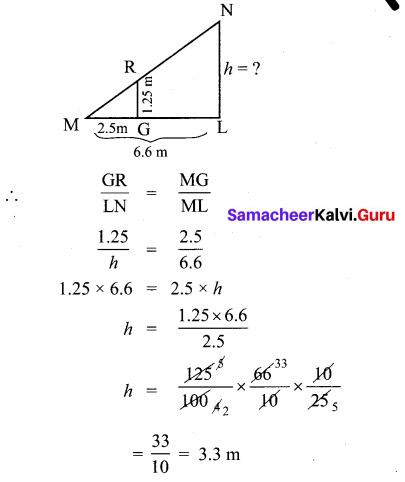∴ Height of the lamp post is 3.3 m.

10th Maths Exercise 4.1 Samacheer Kalvi Question 3.
A vertical stick of length 6 m casts a shadow 400 cm long on the ground and at the same time a tower casts a shadow 28 m long. Using similarity, find the height of the tower.
Solution:
In the picture ∆ABC, ∆DEC are similar triangles.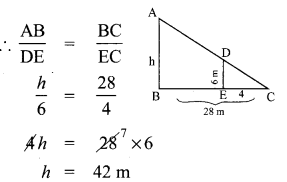Height of a tower = 42 m

Ex 4.1 Class 10 Samacheer Question 4.
Two triangles QPR and QSR, right angled at P and S respectively are drawn on the same base QR and on the same side of QR. If PR and SQ intersect at T, prove that PT × TR = ST × TQ.
Solution:
In ∆RPQ,
RP2 + PQ2 = QR2
∴ PQ2 = QR2 – RP2 ………… (1)
In ∆TPQ,
TP2 + PQ2 = QT2
∴ PQ22 = QT2 – TP2 ………….. (2)
Equating (1) and (2) we get,
QR2 – RP2 = QT2 – TP2
RP = RT + TP
∴ QR2 – (RT + TP)2 = QT2 – TP2
∴ QR2 – RT2 – TP2 – 2RT.TP = QT2 – TP2
QR2 = QT2 + RT2 + 2RT.TP …………. (5)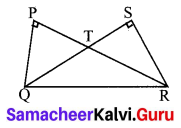In ∆QSR,
QS2 + SR2 = QR2
SR2 = QR2 – SR2 ………..(3)
In ∆TSR,
ST2 + SR2 = TR2
∴ SR2 = TR2 – TS2 ………… (4)
Equating (3) and (4) we get
QR2 – SQ2 = TR2 – TS2
SQ = QT + TS
∴ QR2 – (2T + TS)2 = TR2 – TS2
QR2 – 2T2 – TS2 – 2QT.TS = TR2 – TS2
∴ 2R2 = TR2 + QT2 + 2QT.TS ………… (6)
Now equating (5) – (6), we get
QT2 +RT2 + 2RT. TP = QT2 + RT2 + 2QT.TS
∴ PT.TR = ST.TQ
Hence proved.

10th Geometry Exercise 4.1 Question 5.
In the adjacent figure, ∆ABC is right angled at C and DE⊥AB. Prove that ∆ABC ~ ∆ADE and hence find the lengths of AE and DE?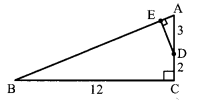Solution:
∠A is common & ∠C = ∠E = 90°
∴ by similarity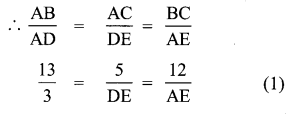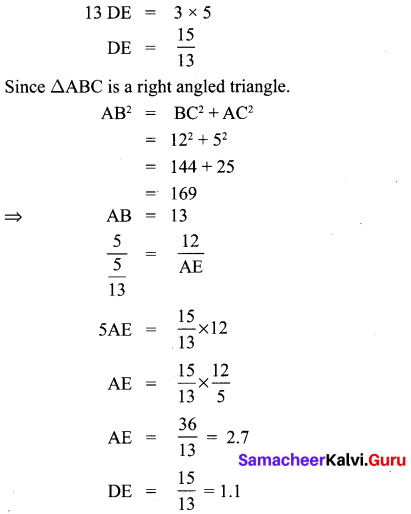Substituting the values of DE and AE in (1)
we can prove that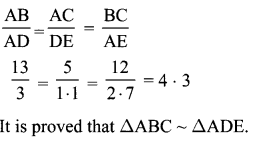Exercise 4.1 Class 10 Samacheer Kalvi Question 6.
In the adjacent figure, ∆ACB ~ ∆APQ . If BC = 8 cm, PQ = 4 cm, BA = 6.5 cm and AP = 2.8 cm, find CA and AQ.Solution:
∆ACB ~ ∆APQ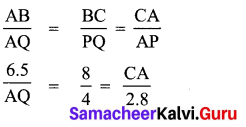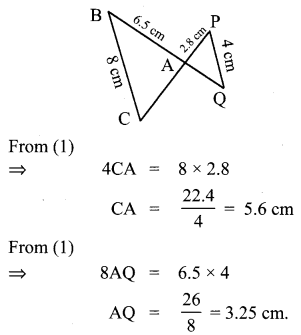10th Maths Geometry Exercise 4.1 Question 7.
In figure OPRQ is a square and MLN = 90°. Prove that
(i) ∆LOP ~ ∆QMO
(ii) ∆LOP ~ ∆RPN
(iii) ∆QMO ~ ∆RPN
(iv) QR2 = MQ × RN.Solution:
(i) In ∆LOP & ∆QMO, we have
∠OLP = ∠MQO (each equal to 90°)
and ∠LOP = ∠OMQ (corresponding angles)
∆LOP ~ ∆QMO (by AA criterion of similarity)

(ii) In ∆LOP & ∆PRN, we have
∠PLO = ∠NRP (each equal to 90°)
∠LPO = ∠PNR (corresponding angles)
∆LOP ~ ∆RPN

(iii) In ∆QMO & ∆RPN .
Since ∆LOP ~ ∆QMO and ∆LOP ~ ∆RPN
∠QMO ~ ∆RPN

(iv) We have
∆QMO ~ ∆RPN (using (iii))
$$\frac{\mathrm{MQ}}{\mathrm{RP}}=\frac{\mathrm{QO}}{\mathrm{RN}}$$ (∵ PROQ is a square)
QR2 = MQ × RN. [RP = QO, QO = QR]

10th Maths Exercise 4.1 In Tamil Question 8.
If ∆ABC ~ ∆DEF such that area of ∆ABC is 9 cm2 and the area of ∆DEF is 16 cm2 and BC = 2.1 cm. Find the length of EF.
Solution:
Since the area of two similar triangles is equal to the ratio of the squares of any two corresponding sides.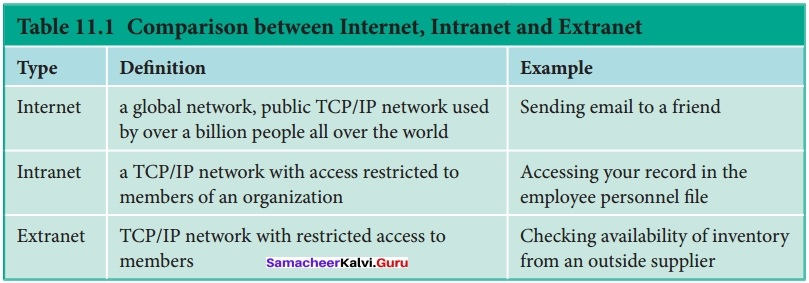10th Maths Exercise 4.1 10th Sum Question 9.
Two vertical poles of heights 6 m and 3 m are erected above a horizontal ground AC. Find the value of y.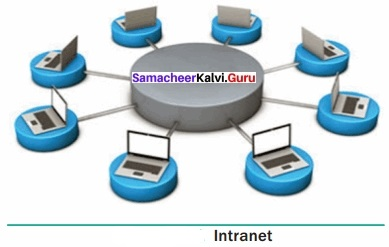Solution:
∆PAC, ∆QBC are similar 6 triangles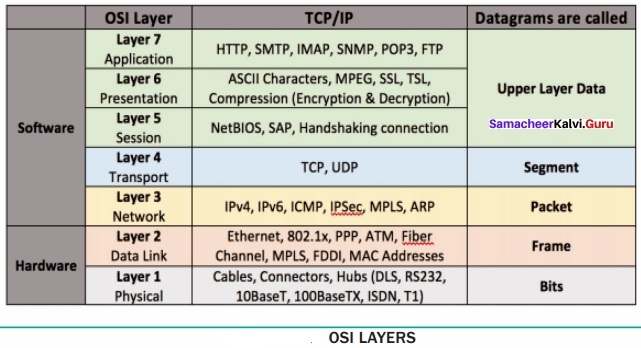⇒ AC = AB + BC
= 2BC + BC
AC = 3BC
Substituting AC = 3BC in (1), we get
(AC)y = 6BC
3(BC)y = 6(BC)
y = $$\frac{6}{3}$$ = 2m

Samacheer Kalvi 10th Maths Practical Geometry Question 10.
Construct a triangle similar to a given triangle PQR with its sides equal to $$\frac{2}{3}$$ of the corresponding sides of the triangle PQR (scale factor $$\frac{2}{3}$$).
Solution:
Given a triangle PQR, we are required to construct another triangle whose sides are $$\frac{3}{5}$$ of the corresponding sides of the triangle PQR.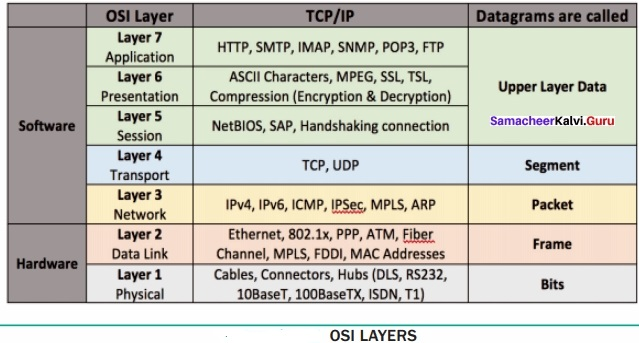Steps of construction:
(1) Draw any ray QX making an acute angle with QR on the side opposite to the vertex P.
(2) Locate 3 (the greater of 2 and 3 in $$\frac{2}{3}$$ ) points. Q1 Q2, Q2 on QX so that QQ1 = Q1Q2 = Q2Q3
(3) Join Q3R and draw a line through Q2 (the second point, 2 being smaller of 2 and 3 in $$\frac{2}{3}$$) parallel to Q3R to intersect QR at R’.
(4) Draw line through R’ parallel to the line RP to intersect QP at P’.
The ΔP’QR’ is the required triangle each of the whose sides is $$\frac{2}{3}$$ of the corresponding sides of 3 ΔPQR.

10th Class Maths Exercise 4.1 Question 11.
Construct a triangle similar to a given triangle LMN with its sides equal to $$\frac{4}{5}$$ of the corresponding sides of the triangle LMN
(scale factor $$\frac{4}{5}$$).
Solution:
Given a triangle LMN, we are required to construct another triangle whose sides are $$\frac{4}{5}$$ of the corresponding sides of the ΔLMN.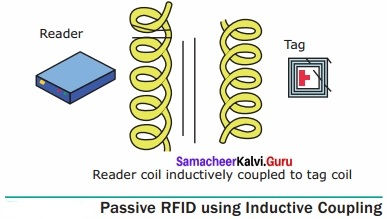Steps of construction:
(1) Draw any ray making an acute angle to the vertex L.
(2) Locate 5 points (greater of 4 and 5 in $$\frac{4}{5}$$ ) M1, M2, M3, M4, and M5 and MX so that MM1 = M1M2 = M2M3 = M3M4 = M4M5
(3) Join M5N and draw a line parallel to M5N through M4 (the fourth point, 4 being the smaller of 4 and 5 in $$\frac{4}{5}$$) to intersect MN atN’.
(4) Draw a line through N1 parallel to the line NL to intersect ML and L’. Then ΔL’MN’ is the required triangle each of the whose sides is $$\frac{4}{5}$$ of the corresponding sides of ΔLMN.

10th Standard Maths Geometry Question 12.
Construct a triangle similar to a given triangle ABC with its sides equal to $$\frac{6}{5}$$ of the corresponding sides of the triangle ABC
(scale factor $$\frac{6}{4}$$ ).
Solution:
ΔABC is the given triangle. We are required to construct another triangle whose sides are $$\frac{6}{5}$$ of the corresponding sides of the given triangle ABC
Steps of construction: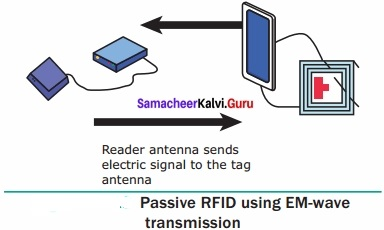(1) Draw any ray BX making an acute angle with BC on the opposite side to the vertex A.
(2) Locate 6 points (the greater of 6 and 5 in $$\frac{6}{5}$$ ) B1, B2, B3, B4, B5, B6 so that BB1 = B1B2 = B2B3 = B4B5 = B5B6.
(3) Join B5 (the fifth point, 5 being smaller of 5 and 6 in $$\frac{6}{5}$$) to C and draw a live through B6 parallel to B5C intersecting the extended line segment BC at C1.
(4) Draw a line through C’ parallel to CA intersecting the extended line segment BA at A’.
Then ΔA’BC’ is the required triangle each of whose sides is $$\frac{6}{5}$$ of the corresponding sides of the given triangle ABC.

10th Maths Exercise 2.1 Samacheer Kalvi Question 13.
Construct a triangle similar to a given triangle PQR with its sides equal to $$\frac{7}{3}$$ of the corresponding sides of the triangle PQR (scale factor $$\frac{7}{3}$$).
Solution:
Given a triangle ΔPQR. We have to construct another triangle whose sides are $$\frac{7}{3}$$ of the corresponding sides of the given ΔPQR.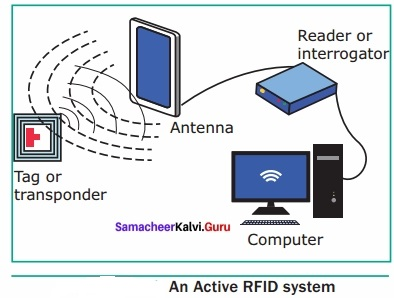Steps of construction:
(1) Draw any ray QX making an acute angle with QR on the opposite side to the vertex P.
(2) Locate 7 points (the greater of 7 and 3 in $$\frac{7}{3}$$) Q1, Q2, Q3, Q4, Q5, Q6, and Q7 so that QQ2 = Q1Q2 = Q2Q3 = Q3Q4 = Q4Q5 = Q5Q6
= Q6Q7
(3) Join Q3 to R and draw a line segment through Q7 parallel to Q3R intersecting the extended line segment QR at R’.
(4) Draw a line segment through R’ parallel to PR intersecting the extended line segment QP at P’.
Then ΔP’QR’ is the required triangle each of whose sides is $$\frac{7}{3}$$ of the corresponding sides of the given triangle.